# Java Program to Determine Number of Bytes Written to File using DataOutputStream

• Difficulty Level : Expert
• Last Updated : 07 Jan, 2021

The Java OutputStream class, java.io.OutputStream, is the base class of all output streams in the Java IO API. An output stream accepts output bytes and sends them to some sink. Applications that need to define a subclass of OutputStream must always provide at least a method that writes one byte of output.

• To get the number of bytes written we firstly create an object of FileOutputStream by passing the file’s path that is shown in code below as FileOS.
• Then we create an object of DataOutputStream by passing the FileOS i.e. object of FileOutputStream that is shown in code below as DataOS.
• Now, we will inject text into this text file by using writeBytes() method of DataOutputStream. To get the size of bytes written we use size method of DataOutputStream Class on the object DataOS.

Program 1:

## Java

 `// Java program to determine number of bytes``// written to DataOutputStream`` ` `import` `java.io.*;`` ` `public` `class` `NumberOfBytesInOutputStream {``    ``public` `static` `void` `main(String[] args)``    ``{``        ``try` `{``           ` `            ``// creates object of FileOutputStream by passing``            ``// file Bytes.txt``            ``FileOutputStream FileOS``                ``= ``new` `FileOutputStream(``"C:/Bytes.txt"``);`` ` `            ``// creates object of DataOutputStream by``            ``// passing object of FileOutputStream i.e.``            ``// FileOS``            ``DataOutputStream DataOS = ``new` `DataOutputStream(FileOS);`` ` `            ``// writes the string passed to object of``            ``// DataOutputStream``            ``DataOS.writeBytes(``                 ``"GeeksforGeeks is the best place to learn Coding online."``);`` ` `            ``// Stores the number of bytes to total_bytes``            ``// variable using size() method of``            ``// DataOutputStream class``            ``int` `total_bytes = DataOS.size();`` ` `            ``// Showing the number of bytes as output in``            ``// console``            ``DataOS.close();`` ` `            ``System.out.println(``"Total "` `+ total_bytes``                        ``+ ``" bytes were written to stream."``);``        ``}``        ``catch` `(Exception e) ``        ``{``            ``System.out.println(``"Exception: "` `+ e.toString());``        ``}``    ``}``}`

Output: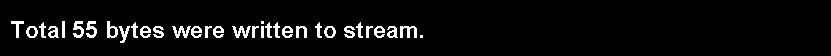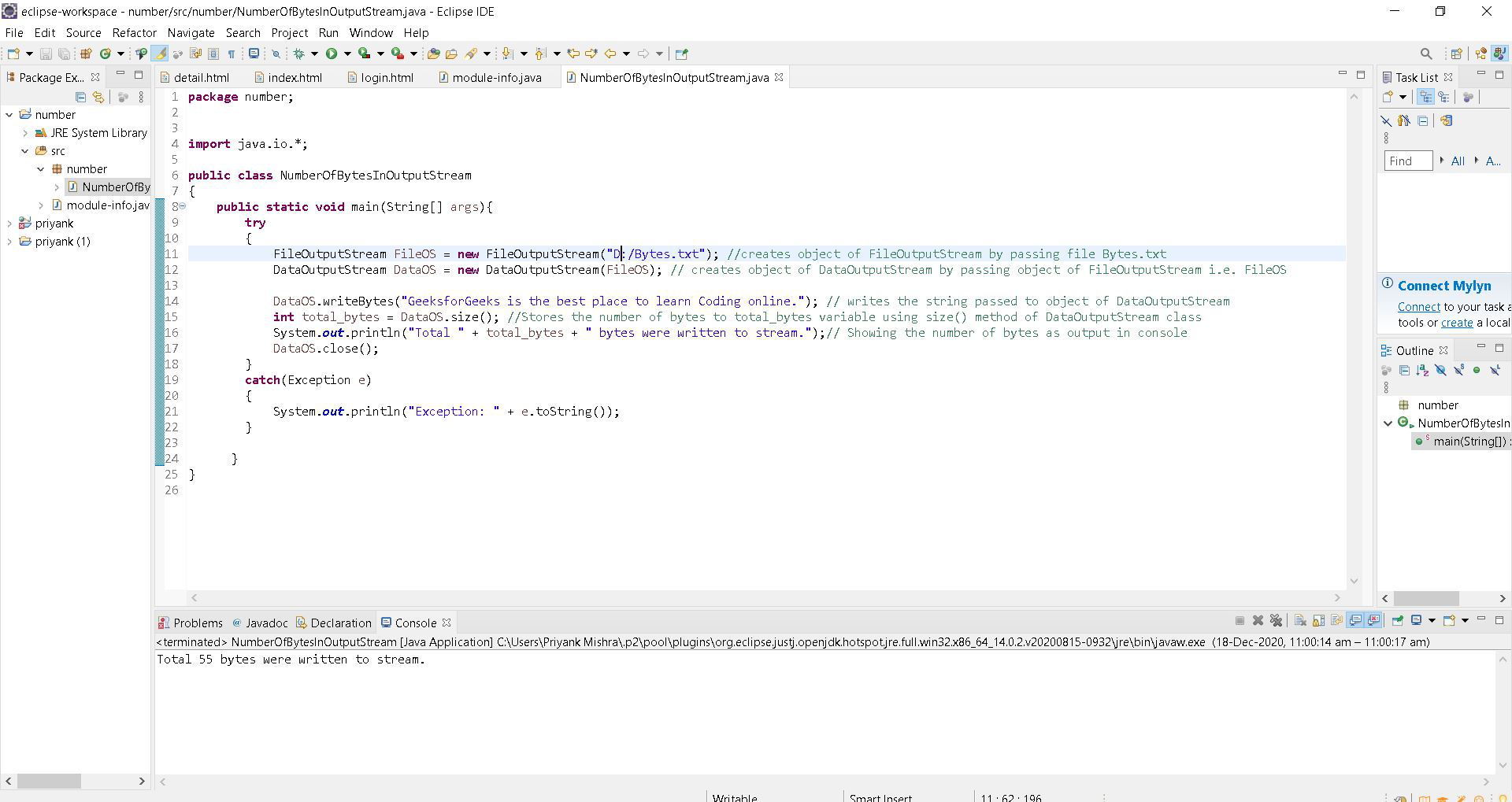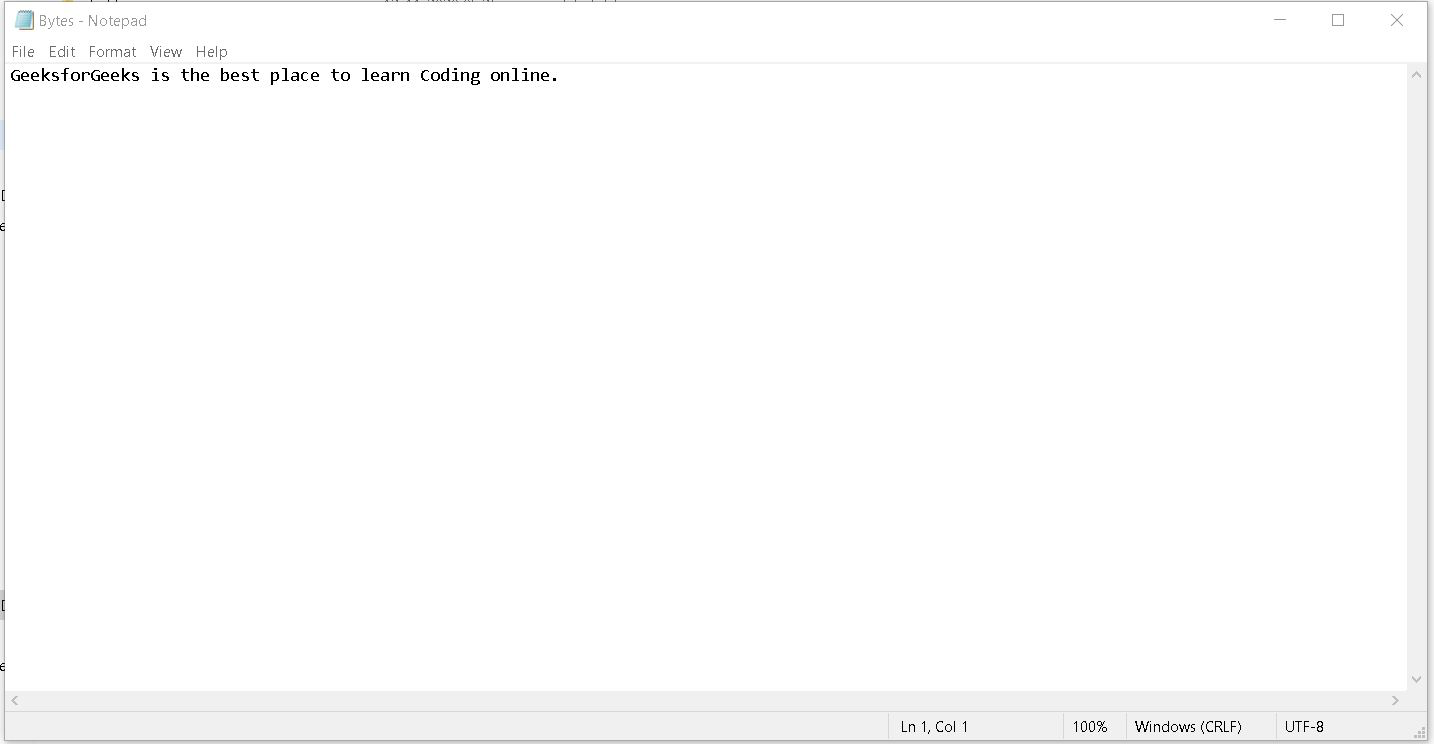Program 2:

## Java

 `// Java program to determine number of bytes``// written to DataOutputStream`` ` `import` `java.io.*;`` ` `public` `class` `NumberOfBytesInOutputStream2 {``    ``public` `static` `void` `main(String[] args)``    ``{``        ``try` `{``           ` `            ``// creates object of FileOutputStream by passing``            ``// file Bytes.txt``            ``FileOutputStream FileOS = ``new` `FileOutputStream(``"C:/NumberOfBytes.txt"``);`` ` `            ``// creates object of DataOutputStream by passing``            ``// object of FileOutputStream i.e. FileOS``            ``DataOutputStream DataOS = ``new` `DataOutputStream(FileOS);`` ` `            ``// create string S with the desired text``            ``String S = ``"GeeksforGeeks article is best for getting cs concepts."``;`` ` `            ``// writes the string passed to object of``            ``// DataOutputStream``            ``DataOS.writeBytes(S);`` ` `            ``// Stores the number of bytes to total_bytes``            ``// variable using size() method of``            ``// DataOutputStream class``            ``int` `total_bytes = DataOS.size();`` ` `            ``// Showing the number of bytes as output in``            ``// console``            ``System.out.println(``"Total "` `+ total_bytes``                      ``+ ``" bytes were written to stream."``);`` ` `            ``DataOS.close();``           ` `        ``}``        ``catch` `(Exception e) ``        ``{``            ``System.out.println(``"Exception: "` `+ e.toString());``        ``}``    ``}``}`

Output: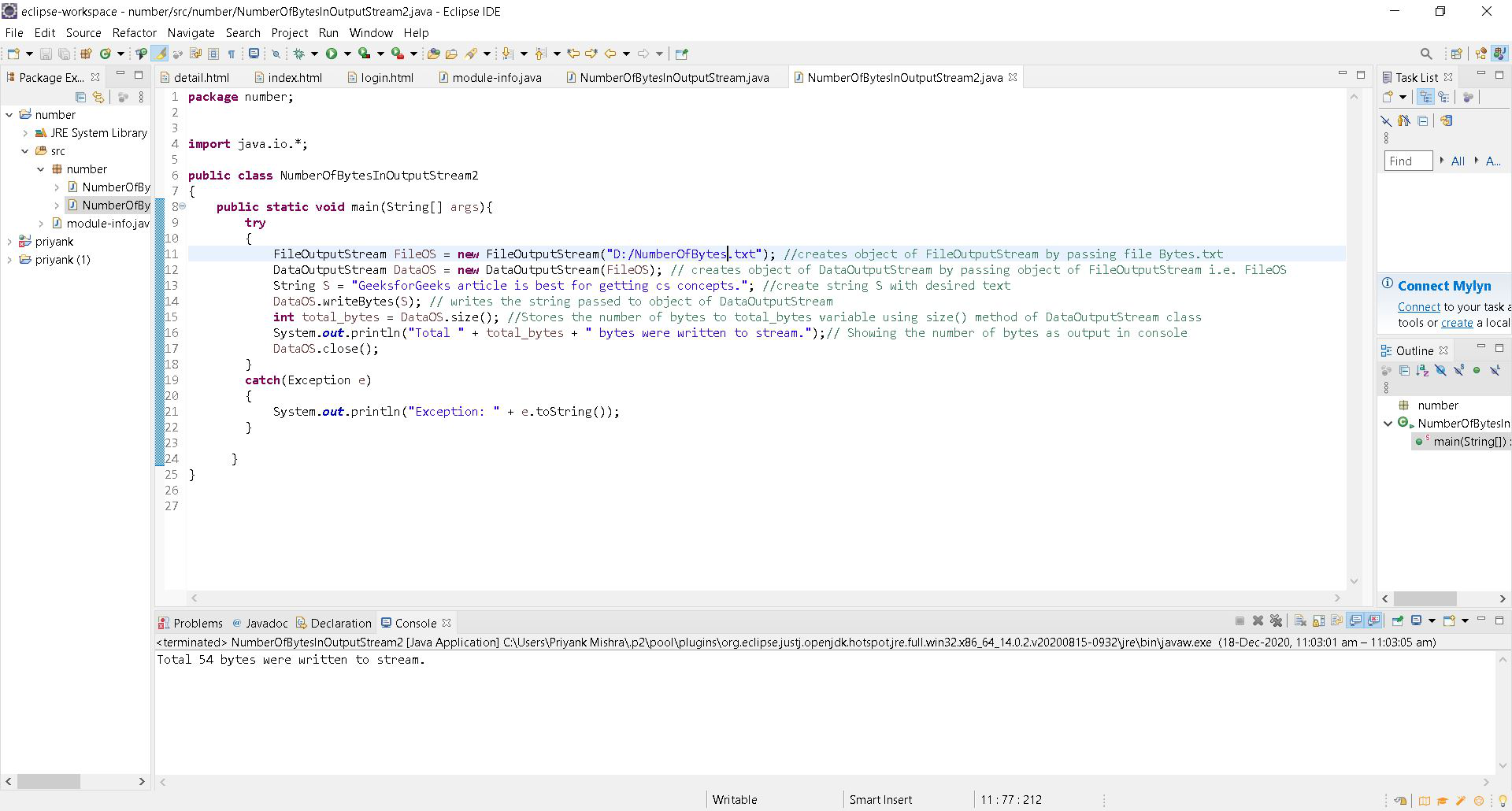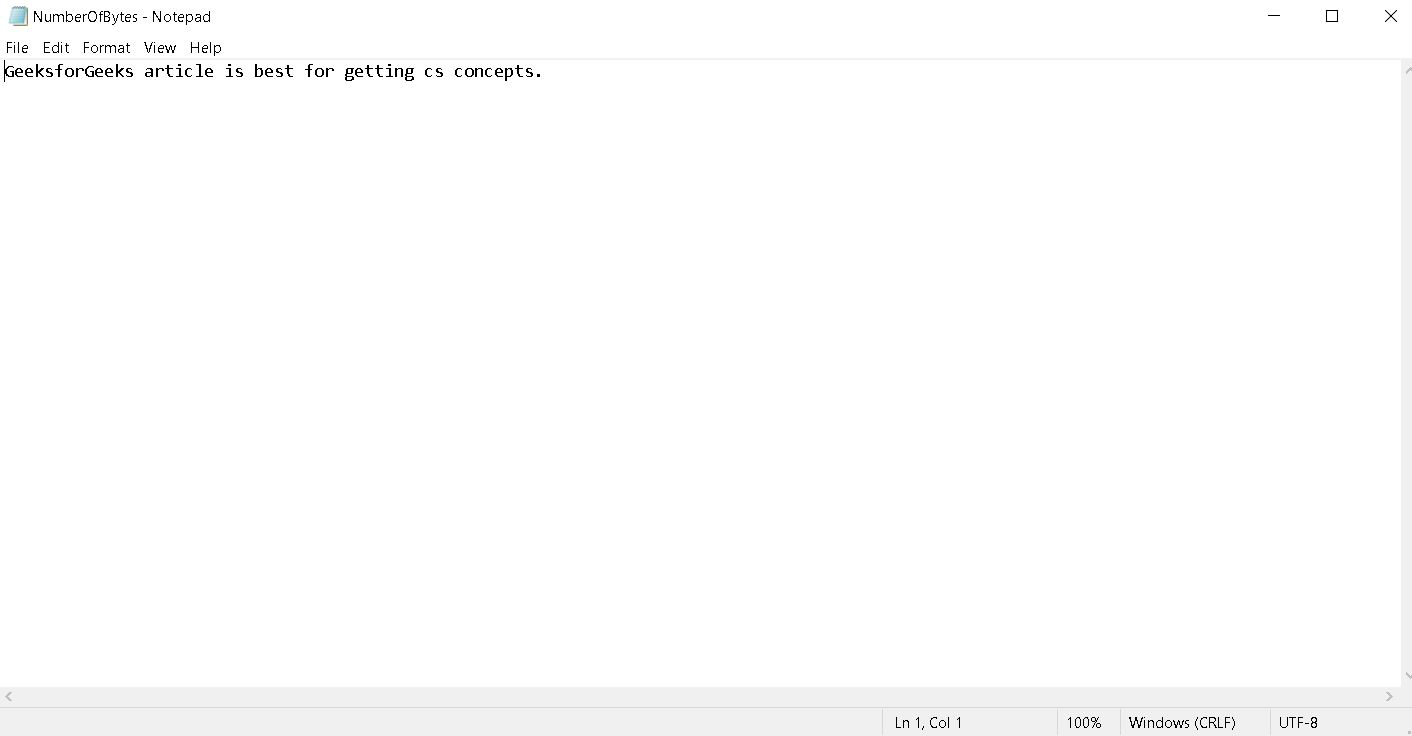My Personal Notes arrow_drop_up